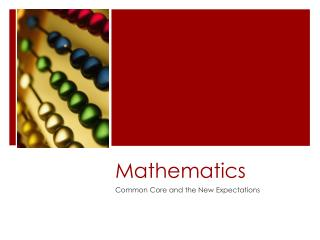DownloadDownload PresentationMathematics

# Mathematics

Télécharger la présentation## Mathematics

- - - - - - - - - - - - - - - - - - - - - - - - - - - E N D - - - - - - - - - - - - - - - - - - - - - - - - - - -
##### Presentation Transcript

1. Mathematics Common Core and the New Expectations

2. Four Corners

3. Think-Pair-Share • Geometry • Computations • Proportional Reasoning • Measurement • Graphing • Probability • Fraction, Decimal, Percent • Equations & Inequalities • Functions • Polynomials Take 3 minutes to read and analyze the problem. In your group, begin discussing what strategies are needed in order to solve the problem. Make a list of the skills needed to solve the problem.

4. Sum-it-up! • Common Core is demanding more from: • Students • Teachers • Word problems that are real world and involve more than just one math concept. • Common Core scoring will be done by super computers. (Hand out answer keys) • www.shawms.weebly.com